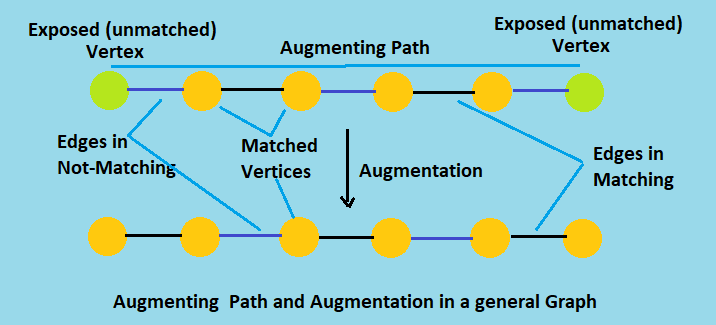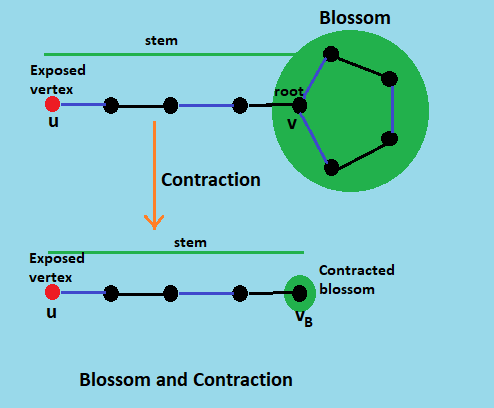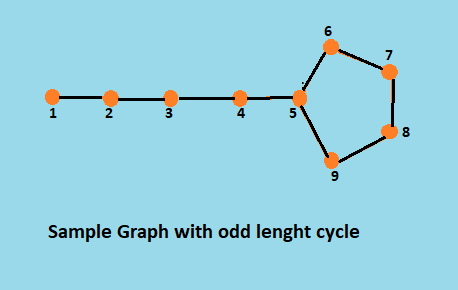×

Search anything:

# Blossom Maximum Matching Algorithm

#### Algorithms Graph Algorithms maximum matchingGet this book -> Problems on Array: For Interviews and Competitive Programming

The blossom algorithm, sometimes called the Edmonds' matching algorithm, can be used on any graph to construct a maximum matching. The blossom algorithm improves upon the Hungarian algorithm by shrinking cycles in the graph to reveal augmenting paths. Additionally, the Hungarian algorithm only works on weighted bipartite graphs but the blossom algorithm will work on any graph.
Given a general graph G = (V, E), the algorithm finds a matching M such that each vertex in V is incident with at most one edge in M and |M| is maximized. The matching is constructed by iteratively improving an initial empty matching along augmenting paths in the graph. Unlike bipartite matching, the key new idea is that an odd-length cycle in the graph (blossom) is contracted to a single vertex, with the search continuing iteratively in the contracted graph.

Augmenting paths
An augmenting path is an odd length path that has unmatched nodes for endpoints. The edges in an augmenting path alternate: one segment will be in the matching, the next segment is not in the matching, the next segment is in the matching, and so on.Blossoms
The idea behind blossoms is that an odd-length cycle in the graph can be contracted to a single vertex so that the search can continue iteratively in the now contracted graph. Here, these odd-length cycles are the blossoms.
A blossom is a cycle in `G` consisting of `2k + 1` edges of which exactly `k` edges belong to matching `M`, and where one of the vertices in the cycle (the base) is such that there exists an alternating path of an even length, called a stem, from `v` to an exposed vertex `u`.# The Blossom Algorithm

The blossom algorithm takes a general graph `G = (V, E)`, and finds a maximum matching `M`. The algorithm starts with an empty matching and then iteratively improves it by adding edges, one at a time, to build augmenting paths in the matching `M`. Adding to an augmenting path can grow a matching since every other edge in an augmenting path is an edge in the matching; as more edges are added to the augmenting path, more matching edges are discovered. The blossom algorithm has three possible results after each iteration. Either the algorithm finds no augmenting paths, in which case it has found a maximum matching; an augmenting path can be found in order to improve the outcome; or a blossom can be found in order to manipulate it and discover a new augmenting path.

``````INPUT:  Graph G, initial matching M on G
OUTPUT: maximum matching M* on G
function find_maximum_matching( G, M ) : M*
P ← find_augmenting_path( G, M )
if P is non-empty then
return find_maximum_matching( G, augment M along P )
else
return M
end if
end function
``````
``````INPUT:  Graph G, matching M on G
OUTPUT: augmenting path P in G or empty path if none found
function find_augmenting_path( G, M ) : P
F ← empty forest
unmark all vertices and edges in G, mark all edges of M
for each exposed vertex v do
create a singleton tree { v } and add the tree to F
end for
while there is an unmarked vertex v in F with distance( v, root( v ) ) even do
while there exists an unmarked edge e = { v, w } do
if w is not in F then
// w is matched, so add e and w's matched edge to F
x ← vertex matched to w in M
add edges { v, w } and { w, x } to the tree of v
else
if distance( w, root( w ) ) is odd then
// Do nothing.
else
if root( v ) ≠ root( w ) then
// Report an augmenting path in F ∪ { e }.
P ← path ( root( v ) → ... → v ) → ( w → ... → root( w ) )
return P
else
// Contract a blossom in G and look for the path in the contracted graph.
B ← blossom formed by e and edges on the path v → w in T
G’, M’ ← contract G and M by B
P’ ← find_augmenting_path( G’, M’ )
P ← lift P’ to G
return P
end if
end if
end if
mark edge e
end while
mark vertex v
end while
return empty path
end function
``````

The goal of the algorithm is to shrinks a blossom into a single node in order to reveal augmenting paths. It works by running the Hungarian algorithm until it runs into a blossom, which it then shrinks down into a single vertex. Then, it begins the Hungarian algorithm again. If another blossom is found, it shrinks the blossom and starts the Hungarian algorithm, and so on.

Each iteration of the inner while loop either adds to a tree T in F or finds an augmenting path or finds a blossom. It is easy to see that the running time is O(E*V2). Each iteration of finding augmented path takes O(E) time, if an augmented path is found, then the matching can be reconfigured in O(V) time, taking O(E*V) time. Lastly, the algorithm of finding maximum matching takes at most O(V) recursions, giving the overall running time of O(E*V2).

# Implementation

Code in C++

``````// C++ Implementation of Edmonds Blossoms' Maximum Matching Algorithm
// It takes Nnumber of vertices and edges, and all the edges of the graph as input
// and gives the maximum matching edges as output

#include <iostream>
#include <cstring>
using namespace std;
#define M 500 // max number of vertices
struct StructEdge
{
int v;
StructEdge *n;
};
typedef StructEdge *Edge;

class Blossom
{
StructEdge pool[M * M * 2];
int V, E, qh, qt;
int match[M], q[M], father[M], base[M];
bool inq[M], inb[M], ed[M][M];
public:
Blossom(int V, int E) : V(V), E(E) {}

{
if (!ed[u - 1][v - 1])
{
ed[u - 1][v - 1] = ed[v - 1][u - 1] = true;
}
}
// utility function for blossom contraction
int LCA(int root, int u, int v)
{
static bool inp[M];
memset(inp, 0, sizeof(inp));
while (1)
{
inp[u = base[u]] = true;
if (u == root)
break;
u = father[match[u]];
}
while (1)
{
if (inp[v = base[v]])
return v;
else
v = father[match[v]];
}
}
void mark_blossom(int lca, int u)
{
while (base[u] != lca)
{
int v = match[u];
inb[base[u]] = inb[base[v]] = true;
u = father[v];
if (base[u] != lca)
father[u] = v;
}
}
void blossom_contraction(int s, int u, int v)
{
int lca = LCA(s, u, v);
memset(inb, 0, sizeof(inb));
mark_blossom(lca, u);
mark_blossom(lca, v);
if (base[u] != lca)
father[u] = v;
if (base[v] != lca)
father[v] = u;
for (int u = 0; u < V; u++)
if (inb[base[u]])
{
base[u] = lca;
if (!inq[u])
inq[q[++qt] = u] = true;
}
}
int find_augmenting_path(int s)
{
memset(inq, 0, sizeof(inq));
memset(father, -1, sizeof(father));
for (int i = 0; i < V; i++)
base[i] = i;
inq[q[qh = qt = 0] = s] = true;
while (qh <= qt)
{
int u = q[qh++];
for (Edge e = adj[u]; e; e = e->n)
{
int v = e->v;
if (base[u] != base[v] && match[u] != v)
if ((v == s) || (match[v] != -1 && father[match[v]] != -1))
blossom_contraction(s, u, v);
else if (father[v] == -1)
{
father[v] = u;
if (match[v] == -1)
return v;
else if (!inq[match[v]])
inq[q[++qt] = match[v]] = true;
}
}
}
return -1;
}
int augment_path(int s, int t)
{
int u = t, v, w;
while (u != -1)
{
v = father[u];
w = match[v];
match[v] = u;
match[u] = v;
u = w;
}
return t != -1;
}
int edmondsBlossomAlgorithm()
{ // Converted recursive algorithm to iterative version for simplicity
int match_counts = 0;
memset(match, -1, sizeof(match));
for (int u = 0; u < V; u++)
if (match[u] == -1)
match_counts += augment_path(u, find_augmenting_path(u));
return match_counts;
}
void printMatching()
{
for (int i = 0; i < V; i++)
if (i < match[i])
cout << i + 1 << " " << match[i] + 1 << "\n";
}

};

int main()
{
int u, v;
int V, E;
cin >> V >> E;
Blossom bm(V, E);
while (E--)
{
cin >> u >> v;
bm.addEdge(u - 1, v - 1);
}
int res = bm.edmondsBlossomAlgorithm();
if(!res)
cout << "No Matching found\n";
else
{
cout << "Total Matching = " << res << "\n";
bm.printMatching();
}
return 0;
}
``````

Sample Input and Output

Sample Graph``````//Input
9 9  // vertices and edges
1 2  // each undirected edge
2 3
3 4
4 5
5 6
6 7
7 8
8 9
9 5
//Output
Total Matching = 4
2 3
4 5
6 7
8 9
``````

# Complexity

Time Complexity

• The Blossoms' algorithm takes O(E*V2) time in worst case.

Space Complexity

• The Blossoms' algorithm takes O(V2) auxiliary space.